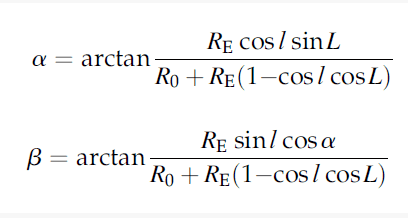# Transform the system of trigonometric equations

• B
• Rmax

#### Rmax

How to extract l and L from the following system of equations:#### Attachments

Please confirm that this is not a homework problem.
First step is to get rid of the arctans in the obvious way. Then taking the ratio you can get an equation relating tan l to sin L. You can use that to eliminate one of them from one of the equations.

Please confirm that this is not a homework problem.
First step is to get rid of the arctans in the obvious way. Then taking the ratio you can get an equation relating tan l to sin L. You can use that to eliminate one of them from one of the equations.
No, it is not a homework.

No, it is not a homework.
Ok, so can you follow the steps I posted?

Ok, so can you follow the steps I posted?
I'll definitely try, thank you!